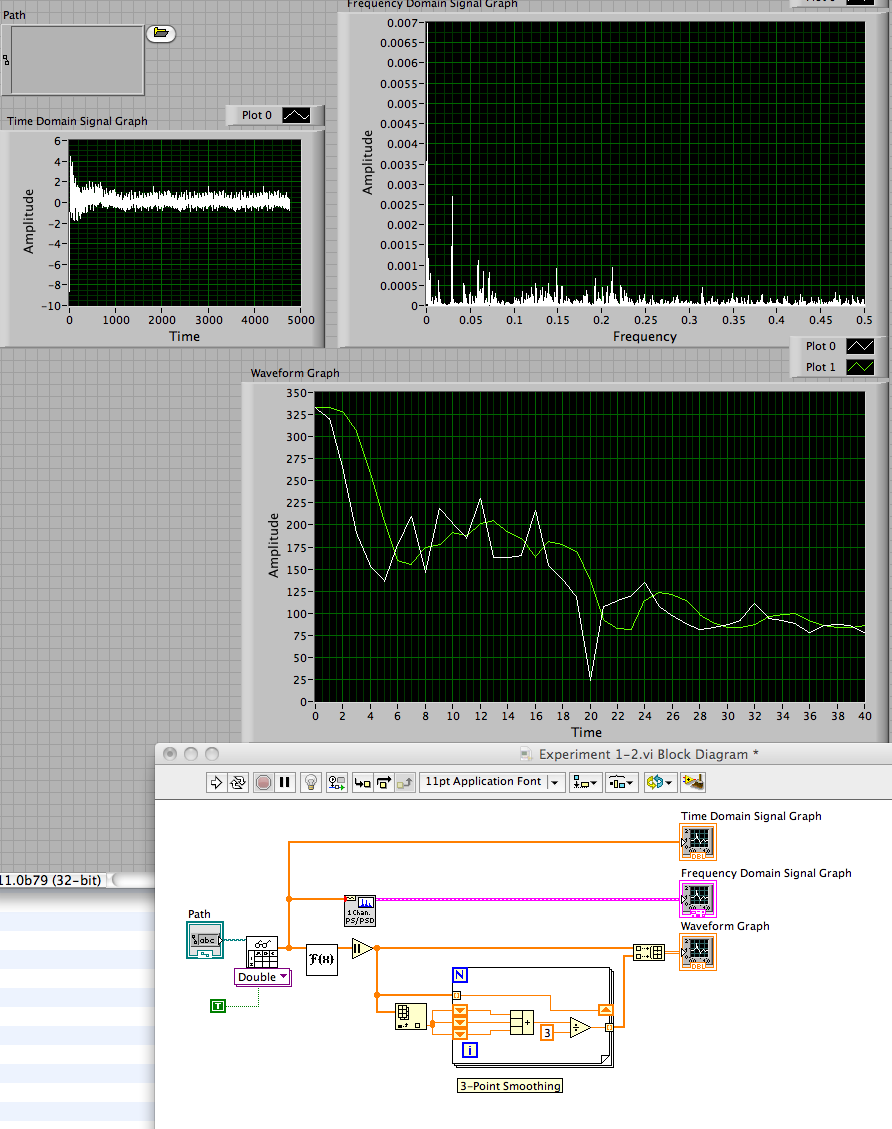# LabVIEW

cancel
Showing results for
Did you mean:

Solved!
Go to solution

## How to reduce noise/smooth out frequency graph

Hello! I have a text file that contains a signal. I had it read in a format of waveforms and plotted the signal in the time domain. I then put the waveform through the PSD vi in order to graph the waveform in the frequency-domain. This graph has a lot of noise in it so I want to have a smoother graph.

One possible way to smooth the graph is to take the average of a few points at each time interval and graph the new values. So I took the waveform signal and split it into x(time) and y(amplitude) arrays. I then took the fourier transform of the amplitude and took the absolute value of it.

From here, I am trying to create a design where I would have the average of the fourier transform values taken every 3 points and then add these points into an array. I would then plot this new array, spaced out evenly between 0 and the end point of the original frequency-domain graph, thereby having a smoother plot.

My design went horribly wrong, however. The resulting graph goes crazy while plotting a straight line and I am also not sure how to have the points evenly spaced out until the original frequency graph's end point. So I have attached my vi and my signal text file and was wondering if anyone could help me solve this problem. Thanks!

Message 1 of 3
(3,885 Views)
Solution

## Re: How to reduce noise/smooth out frequency graph

Yes, it went wrong.  You create a series of arrays of increasing length and constant value.  A 3-point smoothing filter is easy to implement with a shift register.  See the image below.

LynnMessage 2 of 3
(3,875 Views)

## Re: How to reduce noise/smooth out frequency graph

It works perfectly! Thank you!

Message 3 of 3
(3,846 Views)